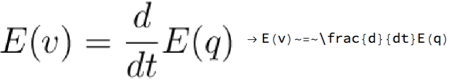#Wolfram Summer School

# Alumni# Himanshu Raj

Science and Technology

Class of 2017

## Bio

I am a PhD student of physics and am currently in the final year of my studies. I have been interested in exploring varied aspects of theoretical high energy physics with particular emphasis on string theory and AdS/CFT correspondence. During my research, I have focused on finding solutions of theories of supergravity and string theory that involve solving coupled PDEs. There are many instances where analytical solutions are not possible to find and one has to make progress via numerical techniques.

I am also interested in applications of artificial neural networks to solve various optimization problems.

## Computational Essay

Spherical Harmonics »

## Project: Image-to-Latex

### Goal of the project:

In this project, we aim to convert any given mathematical expression (printed or handwritten) into LaTeX syntax. We implement the algorithm in the Wolfram Language using the built-in neural network functionality. We follow the algorithm proposed in this paper.

### Summary of work:

The neural network architecture is divided into three stages.

• Convolutional network: The visual features of an image are extracted with a multi-layer convolutional neural network (CNN) interleaved with max-pooling layers. The CNN takes the raw input and produces a feature grid of size D × H × W, where D denotes the number of channels and H and W are the resulting feature map height and width.
• Row encoder: The feature grid produced by the CNN is fed into a row encoder that localizes its input by running a recurrent neural network (RNN) over each of the rows of CNN feature grid and produces a new feature grid V. For OCR, it is important for the encoder to localize the relative positions within the source image.
• Decoder: The target markup tokens {yt} are then generated by a decoder based on the row-encoded feature grid V. The decoder (equipped with an attention mechanism) is trained to calculate the conditional probability of a token [yt+1] appearing at position t+1 given the sequence {y0, y1,…, yt} and the feature V.

### Results and future work:

In this work, we have been able to implement the first two stages of the algorithm and a slightly modified version of the third stage that cuts away the attention mechanism. We have trained the network on a 12 GB NVIDIA GPU. After seven rounds, the test loss drops to 1.48. It is expected that on adding an attention layer in the network, the accuracy should improve.## Wolfram Summer School | Champaign, IL, USA | July 3 29–July 22, 2022

APPLY NOW
• 21st Annual Wolfram Summer School
• Bentley University, Waltham, MA, USA
• June 25–July 15, 2023
• Apply now